dummy-link

81

7

13

11

# NBodySimulatorThis project is under development at the moment. The implementation of potential calculations is fairly experimental and has not been extensively verified yet. You can test simulation of different systems now but be aware of possible changes in the future.

## Add Package

In order to start simulating systems of N interacting bodies, it is necessary to add `NBodySimulator` package to Julia and then begin to use it:

``````]add NBodySimulator
using NBodySimulator
``````

If you cannot wait to start coding, try to run some scripts from the `examples` folder. The number of particles `N` and the final timestep of simulations `t2` are the two parameters which will determine the time of script execution.

## Basic Components

There are three basic components required for any simulation of systems of N-bodies: `bodies`, `system`, and `simulation`.

Bodies or Particles are the objects which will interact with each other and for which the equations of Newton's 2nd law are solved during the simulation process. Three parameters of a body are necessary, namely: initial location, initial velocity, and mass. `MassBody` structure represents such particles:

``````using StaticArrays
r = SVector(.0,.0,.0)
v = SVector(.1,.2,.5)
mass = 1.25
body = MassBody(r,v,mass)
``````

For the sake of simulation speed, it is advised to use static arrays.

A System covers bodies and necessary parameters for correct simulation of interaction between particles. For example, to create an entity for a system of gravitationally interacting particles, one needs to use `GravitationalSystem` constructor:

``````const G = 6.67e-11 # m^3/kg/s^2
system = GravitationalSystem(bodies, G)
``````

Simulation is an entity determining parameters of the experiment: time span of simulation, global physical constants, borders of the simulation cell, external magnetic or electric fields, etc. The required arguments for `NBodySImulation` constructor are the system to be tested and the time span of the simulation.

``````tspan = (.0, 10.0)
simulation = NBodySimulation(system, tspan)
``````

There are different types of bodies but they are just containers of particle parameters. The interaction and acceleration of particles are defined by the potentials or force fields.

## Generating bodies

The package exports quite a useful function for placing similar particles in the nodes of a cubic cell with their velocities distributed in accordance with the Maxwell–Boltzmann law:

``````N = 100 # number of bodies/particles
m = 1.0 # mass of each of them
v = 10.0 # mean velocity
L = 21.0 # size of the cell side

bodies = generate_bodies_in_cell_nodes(N, m, v, L)
``````

Molecules for the SPC/Fw water model can be imported from a PDB file:

``````molecules = load_water_molecules_from_pdb("path_to_pdb_file.pdb")
``````

## Potentials

The potentials or force field determines the interaction of particles and, therefore, their acceleration.

There are several structures for basic physical interactions:

``````g_parameters = GravitationalParameters(G)
m_parameters = MagnetostaticParameters(μ_4π)
el_potential = ElectrostaticParameters(k, cutoff_radius)
jl_parameters = LennardJonesParameters(ϵ, σ, cutoff_radius)
spc_water_paramters = SPCFwParameters(rOH, ∠HOH, k_bond, k_angle)
``````

The Lennard-Jones potential is used in molecular dynamics simulations for approximating interactions between neutral atoms or molecules. The SPC/Fw water model is used in water simulations. The meaning of arguments for `SPCFwParameters` constructor will be clarified further in this documentation.

`PotentialNBodySystem` structure represents systems with a custom set of potentials. In other words, the user determines the ways in which the particles are allowed to interact. One can pass the bodies and parameters of interaction potentials into that system. In the case the potential parameters are not set, the particles will move at constant velocities without acceleration during the simulation.

``````system = PotentialNBodySystem(bodies, Dict(:gravitational => g_parameters, electrostatic: => el_potential))
``````

### Custom Potential

There exists an example of a simulation of an N-body system at absolutely custom potential.

Here is shown how to create custom acceleration functions using tools of NBodySimulator.

First of all, it is necessary to create a structure for parameters for the custom potential.

``````struct CustomPotentialParameters <: PotentialParameters
a::AbstractFloat
end
``````

Next, the acceleration function for the potential is required. The custom potential defined here creates a force acting on all the particles proportionate to their masses. The first argument of the function determines the potential for which the acceleration should be calculated in this method.

``````import NBodySimulator.get_accelerating_function
function get_accelerating_function(p::CustomPotentialParameters, simulation::NBodySimulation)
ms = get_masses(simulation.system)
(dv, u, v, t, i) -> begin custom_accel = SVector(0.0, 0.0, p.a); dv .= custom_accel*ms[i] end
end
``````

After the parameters and acceleration function have been created, one can instantiate a system of particles interacting with a set of potentials which includes the just-created custom potential:

``````parameters = CustomPotentialParameters(-9.8)
system = PotentialNBodySystem(bodies, Dict(:custom_potential_params => parameters))
``````

### Gravitational Interaction

Using NBodySimulator, it is possible to simulate gravitational interaction of celestial bodies. In fact, any structure for bodies can be used for simulation of gravitational interaction since all those structures are required to have mass as one of their parameters:

``````body1 = MassBody(SVector(0.0, 1.0, 0.0), SVector( 5.775e-6, 0.0, 0.0), 2.0)
body2 = MassBody(SVector(0.0,-1.0, 0.0), SVector(-5.775e-6, 0.0, 0.0), 2.0)
``````

When solving a gravitational problem, one needs to specify the gravitational constant G.

``````G = 6.673e-11
``````

Now we have enough parameters to create a GravitationalSystem object:

``````system = GravitationalSystem([body1,body2], G)
``````

Usually, we solve an N-body problem for a certain period of time:

``````tspan = (0.0, 1111150.0)
``````

The created objects determine the simulation we want to run:

``````simulation = NBodySimulation(system, tspan)
sim_result = run_simulation(simulation)
``````

And, finally, we can animate our solution showing two equal bodies rotating on the same orbit:

``````using Plots
animate(sim_result, "path_to_animated_particles.gif")
``````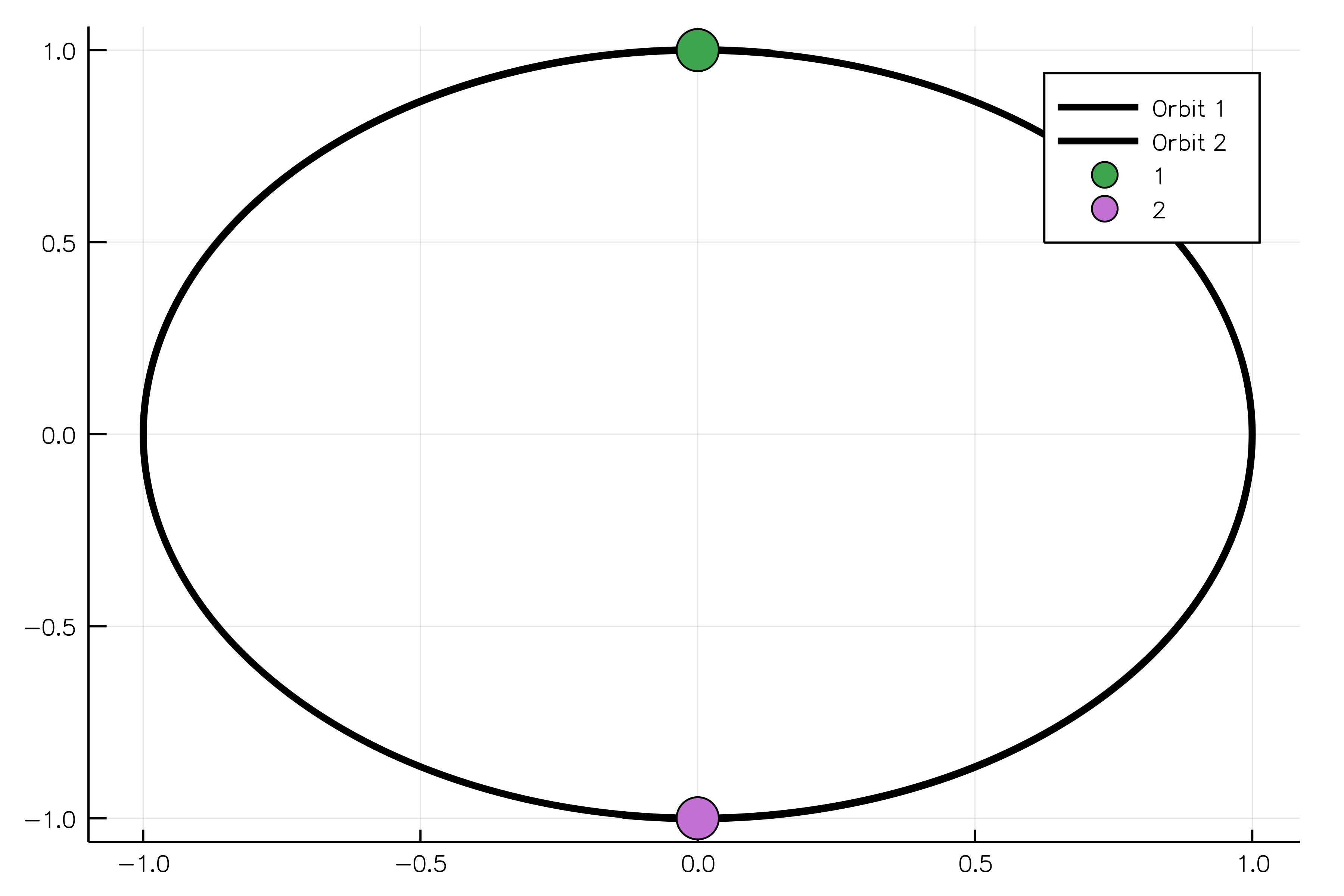### Electrostatic Interaction

Interaction between charged particles obeys Coulomb's law. The movement of such bodies can be simulated using `ChargedParticle` and `ChargedParticles` structures.

The following example shows how to model two oppositely charged particles. If one body is more massive than another, it will be possible to observe rotation of the light body around the heavy one without adjusting their positions in space. The constructor for the `ChargedParticles` system requires bodies and Coulomb's constant `k` to be passed as arguments.

``````r = 100.0 # m
q1 = 1e-3 # C
q2 = -1e-3 # C
m1 = 100.0 # kg
m2 = 0.1 # kg
v2 = sqrt(abs(k * q1 * q2 / m2 / r)) # m/s - using the centrifugal acceleration
t = 2 * pi * r / v2 # s  - for one rotation
p1 = ChargedParticle(SVector(0.0, 0.0, 0.0), SVector(0.0, 0, 0.0), m1, q1)
p2 = ChargedParticle(SVector(100.0, 0.0, 0.0), SVector(0.0, v2, 0.0), m2, q2)
system = ChargedParticles([p1, p2], k)
simulation = NBodySimulation(system, (0.0, t))
sim_result = run_simulation(simulation)
``````

### Magnetic Interaction

An N-body system consisting of `MagneticParticle`s can be used for simulation of interacting magnetic dipoles, though such dipoles cannot rotate in space. Such a model can represent single domain particles interacting under the influence of a strong external magnetic field.

In order to create a magnetic particle, one specifies its location in space, velocity, and the vector of its magnetic moment. The following code shows how we can construct an iron particle:

``````iron_dencity = 7800 # kg/m^3
magnetization_saturation = 1.2e6 # A/m

mass =  5e-6 # kg
r = SVector(-0.005,0.0,0.0) # m
v = SVector(0.0,0.0,0.0) # m/s
magnetic_moment = SVector(0.0, 0.0, magnetization_saturation * mass / iron_dencity) # A*m^2

p1 = MagneticParticle(r, v, mass, magnetic_moment)
``````

For the second particle, we will use a shorter form:

``````p2 = MagneticParticle(SVector(0.005, 0.0, 0.0), SVector(0.0, 0.0, 0.0), 5e-6, SVector(0.0,0.0,0.00077))
``````

To calculate magnetic interactions properly, one should also specify the value for the constant μ0/4π or its substitute. Having created parameters for the magnetostatic potential, one can now instantiate a system of particles which should interact magnetically. For that purpose, we use `PotentialNBodySystem` and pass particles and potential parameters as arguments.

``````parameters = MagnetostaticParameters(μ_4π)
system = PotentialNBodySystem([p1, p2], Dict(:magnetic => parameters))
simulation = NBodySimulation(system, (t1, t2))
sim_result = run_simulation(simulation, VelocityVerlet(), dt=τ)
``````

## Molecular Dynamics (MD)

NBodySimulator allows one to conduct molecular dynamic simulations for the Lennard-Jones liquids, SPC/Fw model of water, and other molecular systems thanks to implementations of basic interaction potentials between atoms and molecules:

• Lennard-Jones
• electrostatic and magnetostatic
• harmonic bonds
• harmonic valence angle generated by pairs of bonds

The comprehensive examples of liquid argon and water simulations can be found in the `examples` folder. Here only the basic principles of the molecular dynamics simulations using NBodySimulator are presented using liquid argon as a classical MD system for beginners.

First of all, one needs to define parameters of the simulation:

``````T = 120.0 # °K
T0 = 90.0 # °K
kb = 8.3144598e-3 # kJ/(K*mol)
ϵ = T * kb
σ = 0.34 # nm
ρ = 1374/1.6747# Da/nm^3
m = 39.95# Da
N = 216
L = (m*N/ρ)^(1/3)#10.229σ
R = 0.5*L
v_dev = sqrt(kb * T / m)
bodies = generate_bodies_in_cell_nodes(N, m, v_dev, L)

τ = 0.5e-3 # ps or 1e-12 s
t1 = 0.0
t2 = 2000τ
``````

Liquid argon consists of neutral molecules so the Lennard-Jones potential runs their interaction:

``````parameters = LennardJonesParameters(ϵ, σ, R)
lj_system = PotentialNBodySystem(bodies, Dict(:lennard_jones => parameters));
``````

Then, a thermostat and boundary conditions should be selected and instantiated:

``````thermostat = NoseHooverThermostat(T0, 200τ)
pbc = CubicPeriodicBoundaryConditions(L)
simulation = NBodySimulation(lj_system, (t1, t2), pbc, thermostat, kb);
result = run_simulation(simulation, VelocityVerlet(), dt=τ)
``````

It is recommended to use `CubicPeriodicBoundaryConditions` since cubic boxes are among the most popular boundary conditions in MD. There are different variants of the `NBodySimulation` constructor for MD:

``````simulation = NBodySimulation(lj_system, (t1, t2));
simulation = NBodySimulation(lj_system, (t1, t2), pbc);
simulation = NBodySimulation(lj_system, (t1, t2), pbc, thermostat);
simulation = NBodySimulation(lj_system, (t1, t2), pbc, thermostat, kb);
``````

The default boundary conditions are `InfiniteBox` without any limits, default thermostat is `NullThermostat` (which does no thermostating), and the default Boltzmann constant `kb` equals its value in SI, i.e., 1.38e-23 J/K.

## Water Simulations

In NBodySImulator the SPC/Fw water model is implemented. For using this model, one has to specify parameters of the Lennard-Jones potential between the oxygen atoms of water molecules, parameters of the electrostatic potential for the corresponding interactions between atoms of different molecules and parameters for harmonic potentials representing bonds between atoms and the valence angle made from bonds between hydrogen atoms and the oxygen atom.

``````bodies = generate_bodies_in_cell_nodes(N, mH2O, v, L)
jl_parameters = LennardJonesParameters(ϵOO, σOO, R)
e_parameters = ElectrostaticParameters(k, Rel)
spc_paramters = SPCFwParameters(rOH, ∠HOH, k_bond, k_angle)
water = WaterSPCFw(bodies, mH, mO, qH, qO,  jl_parameters, e_parameters, spc_paramters);
``````

For each water molecule here, `rOH` is the equilibrium distance between a hydrogen atom and the oxygen atom, `∠HOH` denotes the equilibrium angle made of those two bonds, `k_bond` and `k_angle` are the elastic coefficients for the corresponding harmonic potentials.

Further, one can pass the water system into the `NBodySimulation` constructor as a usual system of N-bodies.

``````simulation = NBodySimulation(water, (t1, t2), pbc, kb);
``````

## Thermostats

Usually, during the simulation, a system is required to be at a particular temperature. NBodySimulator contains several thermostats for that purpose. Here the thermostating of liquid argon is presented, for thermostating of water one can refer to this post

### Andersen Thermostat

``````τ = 0.5e-3 # timestep of integration and simulation
T0 = 90
ν = 0.05/τ
thermostat = AndersenThermostat(90, ν)
``````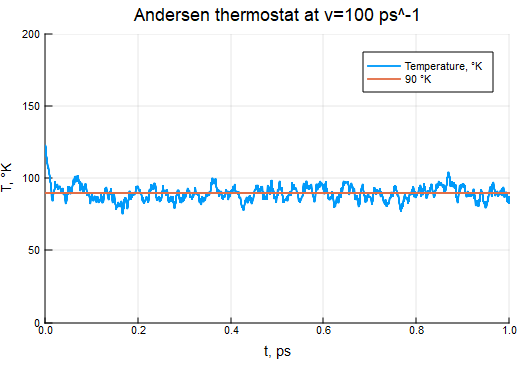### Berendsen Thermostat

``````τB = 2000τ
thermostat = BerendsenThermostat(90, τB)
``````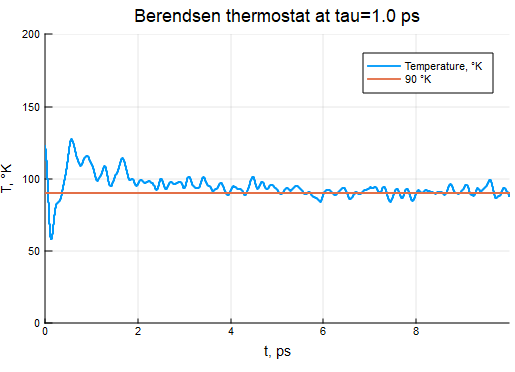### Nosé–Hoover Thermostat

``````τNH = 200τ
thermostat = NoseHooverThermostat(T0, 200τ)
``````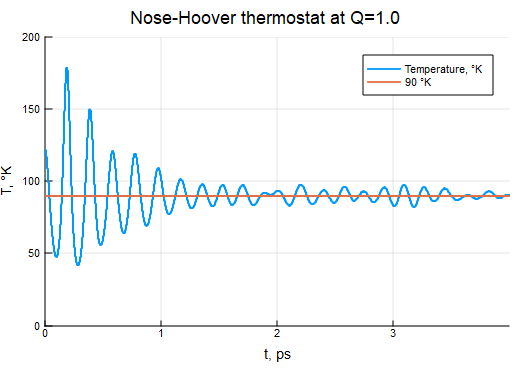### Langevin Thermostat

``````γ = 10.0
thermostat = LangevinThermostat(90, γ)
``````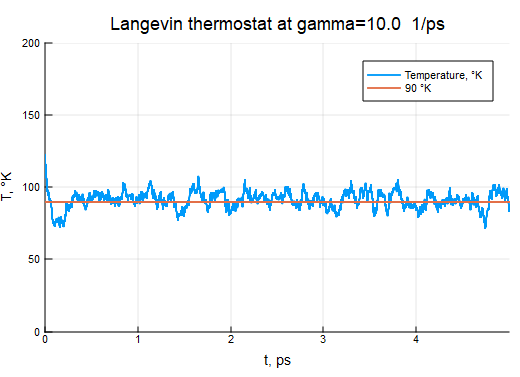## Analyzing the Result of Simulation

Once the simulation is completed, one can analyze the result and obtain some useful characteristics of the system.

The function `run_simulation` returns a structure containing the initial parameters of the simulation and the solution of the differential equation (DE) required for the description of the corresponding system of particles. There are different functions which help to interpret the solution of DEs into physical quantities.

One of the main characteristics of a system during molecular dynamics simulations is its thermodynamic temperature. The value of the temperature at a particular time `t` can be obtained via calling this function:

``````T = temperature(result, t)
``````

### Radial distribution functions

The RDF is another popular and essential characteristic of molecules or similar systems of particles. It shows the reciprocal location of particles averaged by the time of simulation.

``````(rs, grf) = rdf(result)
``````

The dependence of `grf` on `rs` shows radial distribution of particles at different distances from an average particle in a system. Here the radial distribution function for the classic system of liquid argon is presented: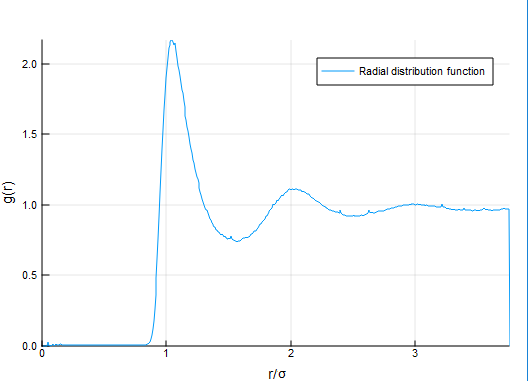### Mean Squared Displacement (MSD)

The MSD characteristic can be used to estimate the shift of particles from their initial positions.

``````(ts, dr2) = msd(result)
``````

For a standard liquid argon system, the displacement grows with time: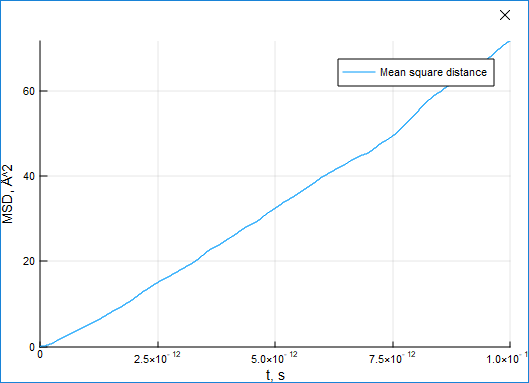### Energy Functions

Energy is a highly important physical characteristic of a system. The module provides four functions to obtain it, though the `total_energy` function just sums potential and kinetic energy:

``````e_init = initial_energy(simulation)
e_kin = kinetic_energy(result, t)
e_pot = potential_energy(result, t)
e_tot = total_energy(result, t)
``````

## Plotting Images

Using tools of NBodySimulator, one can export the results of a simulation into a Protein Database File. VMD is a well-known tool for visualizing molecular dynamics, which can read data from PDB files.

``````save_to_pdb(result, "path_to_a_new_pdb_file.pdb" )
``````

In the future it will be possible to export results via FileIO interface and its `save` function.

Using Plots.jl, one can draw positions of particles at any time of simulation or create an animation of moving particles, molecules of water:

``````using Plots
plot(result)
animate(result, "path_to_file.gif")
``````

Makie.jl also has a recipe for plotting the results of N-body simulations. The example is presented in the documentation.

07/24/2018

8 days ago

92 commits